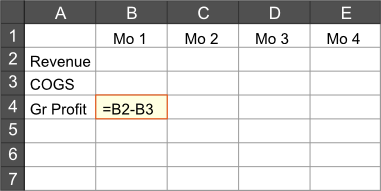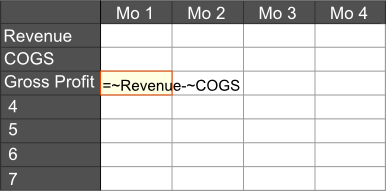Not Just Cells

When you are building financial models, many times you want to repeat a formula across a range of cells. The way you normally do this in a spreadsheet is to copy the 1st formula and then paste that copied formula to the cells. For example, if you were building a model such as the following:You would normally copy the cell highlighted in orange (cell B4) and then paste it into the subsequent cells (C4:E4). This allows you to easily calculate gross profit for months 1 through 4 and you are on your way. Two questions immediately arise though:

1. Why do you have to use copy / paste to get the desired result?
2. What do you do when you want to add month 5 (and months 6, 7, etc.)?

You'll notice that the 1st question, while not a huge challenge, is a little bit strange. But it actually becomes more significant in the context of the 2nd question. While the examples may seem a bit contrived, the challenges faced are real. It is inherent to the modeling process - you almost always need the flexibility to start the modeling process on something small with the intention of growing the model as you get more details and need to be more precise.

Thus, one of the things that we've been working on for the Grid Mule is giving you the ability to define formulas not only in terms of cell references (like A1), but also in terms of rows or columns (like column A or row 1, separately). We also give you ability to rename columns and rows. You could therefore change the name of row 1 to Revenue, row 2 to COGS and row 3 to Gross Profit. You could also change the names of the columns from A to Mo 1, B to Mo 2 and so on. You could then enter a formula in Mo 1~Gross Profit (formerly A1) of =~Revenue-~COGS like so:and it would work just like you expect. And whenever you add a value to the end of the vector (e.g., adding 'Mo 5'), the formula will automatically follow through to that cell.

The ~ (tilde) character is necessary because we are referencing a row that doesn't follow the "normal conventions" (viz., it's not a simple number). Thus, the tilde is used to denote the separation of a column and a row. Since we don't wish to specify a column, we leave it blank / empty. If we wanted to obtain the revenue for month 1, the reference would be Mo 1~Revenue.

At some point we hope to add the ability to define formulas for custom ranges (e.g., instead of requiring a formula for an entire row or column, define it for a subset of cells like A3:D3 = ~Revenue-~COGS). But for now, we think this is an improvement over the current status quo. We hope you think so to.